• 2022-04-11 16:06:50

### 一、自定义函数的固定语句

def contrast(a,b) :      #使用def来定义一个名称为contrast的方法,a与b的值是两个变量，称为形参
if a>b :       #使用条件语句进行判定
return a           #返回a的值
elif b>a :
return b           #返回b的值
else:
return ('a=b')    #返回字符串a=b

c = contrast(1,1)     #实例化方法contrast
print(c)           #输出c

输出结果：

a=b

可以引用随机数进行判定：

import random       #引用随机函数
x = random.randint(1,5)    #使用随机函数中的随机整数方法
y = random.randint(1,5)
c = contrast(x,y)         #调用contrast方法，这时变量x和y,称为实参
print(x,y,c)

输出结果：

1 1 a=b

### 二、模块化自定义代码的引用

一般我们写好某些自定义函数方法后存储在.py文件中，需要的时候我们直接调用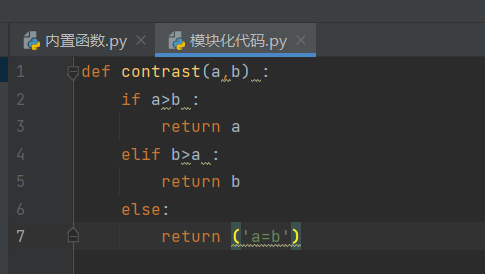创建一个.py结尾的文件，并存入你自己定义的方法函数

import random
from 模块化代码 import *      #从模块化代码文件中引用所有方法，引用指定方法就写方法名称就可以
x = random.randint(1,5)
y = random.randint(1,5)
c = contrast(x,y)
print(x,y,c)

运行结果：

2 5 5

注意：*为所有方法，当我们储存自定义方法函数的文件和Python运行代码不在同一个目录，则需要写上绝对路径，例如：方法位置为：pythonProject/模块化代码.py，我们调用时需要注明路径为：from pythonProject.模块化代码 import *

### 三、可变变量和不可变变量

不可变变量

def f(a) :       #定义方法f()
a = 'boy'
return a

b = 'girl'
c = f(b)         #实例化方法f()
print(c)

输出结果：

boy

注意：字符串是不可变变量，这样操作无意义

可变变量

def f(a) :          #定义方法f()
a.append('boy')     #追加字符串
return a

b = ['girl']
c = f(b)    #实例化方法f()
print(c)

输出结果：

['girl', 'boy']     #列表是可变的，使用追加方法。

### 四、自定义函数方法中的避免被另一个文件调用的语句

def contrast(a,b) :
if a>b :
return a
elif b>a :
return b
else:
return ('a=b')
if __name__ == '__main__' :  #写在这个语句下的所有代码都不会被调用，只在本文件中运行
def f(a):
a.append('boy')
return a
b = ['girl']
c = f(b)
print(c)python
更多相关内容
• 引荐教程：《python视频教程》python自定义函数实例有哪些？python自定义函数实例有：1、什么是函数？函数是构造好的，可反复使用的，用来完成单一，或相干联功用的代码段。函数能进步运用的模块性，和代码的反复...引荐教程：《python视频教程》

python自定义函数实例有哪些？

python自定义函数实例有：

1、什么是函数？

函数是构造好的，可反复使用的，用来完成单一，或相干联功用的代码段。函数能进步运用的模块性，和代码的反复利用率。

2、函数的定义要领：

def test(x):

'函数定义要领'

x+=1

return x

诠释：

def：定义函数症结字

test：函数名

()：可定义形参

''：文档形貌

x+=1:代码块或程序处置惩罚逻辑

return:完毕并返回值

函数为何要有返回值？

经由过程返回值吸收函数的实行效果，后续的逻辑须要经由过程此效果实行其对应操纵。

(1)、实例：给一个文件写入日记信息

import time

# 定义函数

def test1():

'函数演习:增加日记纪录'

log_time = time.strftime('%Y-%m-%d %X')

with open('file_a','a') as f:

f.write(log_time+':log msg\n')

# 挪用函数

test1()

(2)、函数返回值申明：

return 返回值数目=0：返回一个空值(None)

返回值数目=1：返回一个对象(object)

返回值数目>1：返回一个元组(tuples)

实例：

#函数返回范例

def test_None():

print('返回一个空值')

x=test_None()

print(x)

def test_object():

print('返回一个对象')

return 0

y=test_object()

print(y)

def test_tuples():

print('返回一个元组')

return 1,'hello world',['qwe','asd'],{'001':'simple'}

z=test_tuples()

print(z)

(3)、形参：定义的参数叫形参(x,y)

实参：现实传入的参数叫实参(1,2)

不指定参数的情况下：实介入形参位置一一对应

实例：

注：位置传介入症结字传参共用时，症结字参数不能写在位置参数之前

def test_sum(x,y):

'两数之和'

z = x + y

return z

t_sum=test_sum(1,2) #实介入形参位置一一对应

print(t_sum)

t_sum2=test_sum(x=1,y=2) #与形参位置无关

print(t_sum2)

t_sum3=test_sum(1,y=2) # 毛病体式格局：test_sum(x=1,2) 位置传介入症结字传参共用时，症结参数不能写在位置参数之前

print(t_sum3)

(4)、默许值参数：

# 默许值参数

def test_default(x,y=2):

'默许值参数'

z = x + y

return z

print(test_default(2)) #print(tesst_default(2,2))

(5)、参数组(不定长参数)：

# 参数组,接收位置参数，将多个实参存入一个元组中

# 定义花样：*变量名(平常范例为 *args)

def test_group(*args):

'参数组'

print(args)

test_group(1,2,3,4,5,6)

def test_group2(x,*args):

'参数组与位置参数混用'

print(x)

print(args)

test_group2(1,2,3,4,5,6)

# 接收症结字参数组，转换成字典

def test_group3(**kwargs):

'参数组键值对情势'

print(kwargs['name'])

test_group3(name='simple',age=25,sex='m')

def test_group4(name,**kwargs):

'参数、参数组键值对混用'

print(name)

print(kwargs)

test_group4('simple',age=25,sex='m')

#注：参数组必需放在位置参数、默许参数以后

def test_group5(name,age=25,**kwargs):

'参数、默许参数、参数组键值对混用'

print(name)

print(age)

print(kwargs)

test_group5('simple',age=3,sex='m',game='lol')

def test_group6(name,age=25,*args,**kwargs):

'参数、默许参数、参数组、参数组键值对混用'

print(name)

print(age)

print(*args)

print(kwargs)

test_group5('simple',age=3,sex='m',game='lol')

引荐相干文章：《python教程》

以上就是python自定义函数实例有哪些？的细致内容，更多请关注ki4网别的相干文章！

收藏 | 0

展开全文• 在本文里我们给大家整理了关于python调用自定义函数实例操作相关内容，有此需要的朋友们可以学习参考下。
• 但你也可以自己创见函数，这被叫做用户自定义函数。一、定义一个函数你可以定义一个由自己想要功能的函数，以下是简单的规则： 1.函数代码块以def关键词开头，后接函数标识符名称和圆括号()。2.任何传入参数和自变量...
• python学习之自定义函数

python学习之自定义函数
一、不带参数函数
不带参数函数格式

def 函数名():
函数体


不带参数函数的一个案例

def _factor():    #定义函数
i=1
nums=10
print("%d的因数为:"%(nums))
while i<=nums:    #循环求因数
if nums%i==0:
print('%d'%i)
i+=1
#===========================
_factor()   #调用函数
t=type(_factor)  #检查是否是函数类型
print(t)

'''结果为:
10的因数为:
1
2
5
10
<class 'function'>  #是函数类型
'''



注意：使用自定义函数，必须先定义或先引入定义模块文件，再调用自定义函数。不能先调用，再定义，否则报“找不到自定义函数错误”。

二、带参数的函数
带参数函数格式

def 函数名(参数):
函数体


带参数函数案例
如：

def find_factors(nums):
i = 1
str1 = ''
print("%d的因数为:" % nums)
while i < nums:
if nums % i == 0:
str1 = str1 + ' ' + str(i)   #用字符串记录一个整数的因数
i += 1
print(str1)

# =======================================
num_list = [10, 18, 20, 30]
i = 0
num_length = len(num_list)
while i < num_length:
find_factors(num_list[i])
i += 1



与不带参数的函数相比，更加灵活，实用，可以接受任意正整数的传递并求因数。

通常把def 函数名(参数)的参数叫形式参数，调用函数时赋予的值叫实际参数。

三、带返回值的函数
格式：

def 函数名([参数]):
函数体
return 返回值


函数返回值：不带return语句的函数，其实默认都返回None值，如调用_factor()（不带参数案例），

def _factor():  # 定义函数
i = 1
nums = 10
print("%d的因数为:" % (nums))
while i <= nums:  # 循环求因数
if nums % i == 0:
print('%d' % i)
i += 1

# ===========================
# _factor()  # 调用函数
print(_factor())
# t = type(_factor)  # 检查是否是函数类型
# print(t)
'''结果为：
10的因数为:
1
2
5
10
None'''


return语句在函数中除了返回值外，还起中断函数执行作用。
如：

def test():
return 1  #return 将终止函数执行并返回值1
print("Yes") #该语句不会执行
print(test())


带返回值的函数的案例（求因数）

def find_factors(nums):
i = 1
str1 = ''
while i < nums:
if nums % i == 0:
str1 = str1 + ' ' + str(i)   #用字符串记录一个整数的因数
i += 1
# print(str1)
return str1

# =======================================
num_list = [10, 18, 20, 30]
i = 0
num_length = len(num_list)
return_str=''
while i < num_length:
return_str=find_factors(num_list[i]) #循环调用函数，并返回因数字符串
print("%d的因数为：%s"%(num_list[i],return_str))
i += 1


结果为：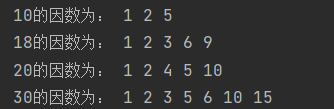该案例的好处：
1、可以灵活获取处理结果，为调用代码所用
2、把附加的打印功能转移到函数体外，提高了调用者处理打印信息的灵活性。

四、自定义函数的完善

def find_factors(nums):
'''
find_factors是自定义函数
nums是传递正整数的参数
以字符串形式返回一个正整数的所有因数'''  #用一对三个单引号来包括描述文档
i = 1
str1 = ''
while i < nums:
if nums % i == 0:
str1 = str1 + ' ' + str(i)   #用字符串记录一个整数的因数
i += 1
# print(str1)
return str1

help(find_factors) #help函数用来获取函数的使用功能的信息。



可以得到以下结果：得到函数的相关信息，是用一对三个单引号的描述文档的内容。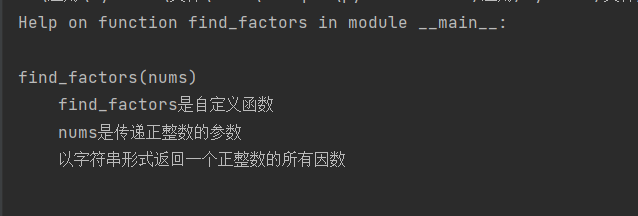健壮性考虑

函数的参数是传递值的入口，必须考虑所传递的值是否符合要求。
如向find_factors(nums)传递一个字符，一个符号
如find_factors(‘a’)，
会出现
TypeError: ‘<’ not supported between instances of ‘int’ and ‘str’
错误。

实际应用中出现这样的错误会非常糟糕，所以要对函数进一步完善。

我们需要在函数中进行如下完善：

if type(nums)!=int:
print('输入值类型出错，必须是整数')
return
if nums<=0:
print('输入值范围出错，必须是正整数')
return


五、把函数放到模块中
自定义函数建立后，如果需要被其他代码文件调用（以.py为扩展名的文件），或者需要通过共享，让其他程序员使用，需要把函数代码单独存放到一个可以共享的地方。
在Python语言中，通过建立独立的函数模块（Module）文件（以.py为扩展名的文件），共享给其他代码文件调用。
1、建立函数模块
1）首先新建一个空白的代码文件，存放自定义函数代码，如建立一个test.py的空函数模块文件。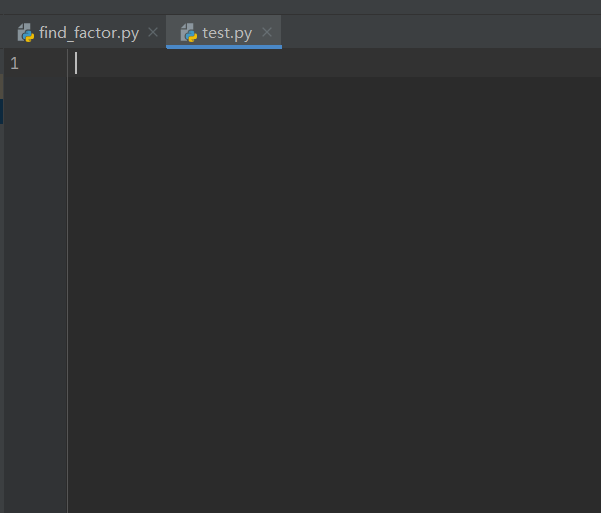2）编写并调试完成自定义函数代码，如前面的find_factors(nums)函数。
3）把只属于自定义函数的代码复制到函数模块文件上，若有多个自定义函数，按照顺序复制即可。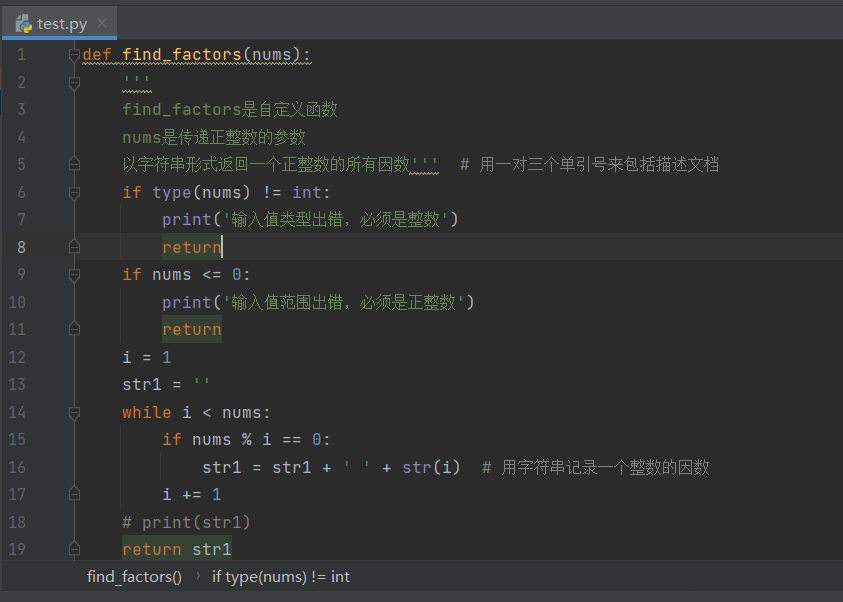test.py函数模块文件，实现了对find_factors(nums)函数代码的完整保存，完成这个过程后，该函数被其他python文件中的代码进行调用成了可能。

2、调用函数模块
在python语言编辑器里，除了默认内置函数外，其他函数的调用，必须先通过import语句进行导入，才能使用。

1）import语句导入整个函数模块
格式：import 函数模块名
（1）新建一个python文件，文件中输入调用代码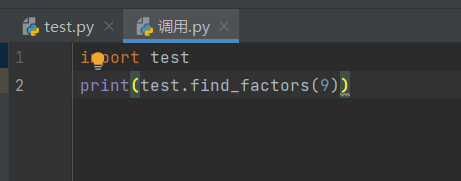（2）执行后结果为：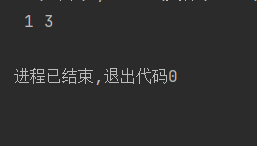import语句直接导入模块后，调用模块文件里的函数格式如下：
模块名.函数名
通过模块名中间连接点号与函数名连接方式调用函数。
注意：必须要保证 调用.py 和模块文件test.py在同一个文件夹下，不然会报“找不到模块错误(ModuleNotFoundError)”。

2）import导入指定函数
格式：from 模块名 import 函数名1[,函数名2,…]

# import test
# print(test.find_factors(9))
from test import find_factors
print(find_factors(9))


该方法可以像内置函数一样使用模块中的自定义函数。

3）import导入所以函数
格式：from 模块名 import *
"*"代表指定模块里的所有函数。

4）模块名、函数名别名方式
如果导入模块，函数时发现名称过长，可以通过as定义别名的方式解决。
格式：模块名[函数名] as 别名

import test as t #给模块名定义别名

print(t.find_factors(9))

from test import find_factors as f #为自定义函数定义别名

print(f(9))



as语句还可以解决函数名称发生冲突的问题。

3、模块搜索路径
主要是解决在不同文件夹下模块调用的访问路径的问题，不然会报“找不到模块”的错误。

用sys.path方法指定需要访问的函数模块文件。

import sys

sys.path = 'C:\应用\Pycharm\文件\\function'  # 模块路径
from test import find_factors

print(find_factors(10))
# 结果为：
#  1 2 5


正常的路径格式为’C:\应用\Pycharm\文件\function’格式，当时”\f“为”换页“转义字符，所以为了得到正确的路径，要在”\f“前在加一个”\“,让’\f‘原样输出。

展开全文开发语言 后端
• 今天小编就为大家分享一篇python自定义函数实现最大值的输出方法，具有很好的参考价值，希望对大家有所帮助。一起跟随小编过来看看吧
• filter()函数可以对序列做过滤处理，就是说可以使用一个自定的函数过滤一个序列，把序列的每一项传到自定义的过滤函数里处理，并返回结果做过滤。最终一次性返回过滤后的结果。 filter()函数有两个参数： 第一个，自...
• python自定义函数在运行时，最初只是存在内存中，只有调用时才会触发运行。 def cube_count(a): if is_number(a): return a**3 else: print(非数字不能计算立方值) def is_number(a): if not isinstance(a,...
• 本文实例讲述了Python学习笔记之自定义函数用法。分享给大家供大家参考，具体如下： 函数能提高应用的模块性，和代码的重复利用率。Python提供了许多内建函数，比如print()等。也可以创建用户自定义函数。 函数定义 ...
• 本文实例讲述了Python自定义函数实现求两个数最大公约数、最小公倍数。分享给大家供大家参考，具体如下： 1. 求最小公倍数的算法: 最小公倍数 = 两个整数的乘积 / 最大公约数 所以我们首先要求出两个整数的最大公...
• Python中，函数有内置函数和自定义函数2种。 内置函数是指：像print、input、type 等安装Python后就能直接使用的函数。 自定义函数是指：根据自己想要实现的功能自定义一个属于自己的函数。 函数具有函数名、参数、...

# 第七章 函数

## 7.1 自定义函数的创建及调用

函数是指编写好的，可以重复使用的代码段。
Python中，函数有内置函数和自定义函数2种。
内置函数是指：像print、input、type 等安装Python后就能直接使用的函数。
自定义函数是指：根据自己想要实现的功能自定义一个属于自己的函数。
函数具有函数名、参数、返回值。

体验代码：计算矩形的面积
先运行下面的代码，体验自定义函数的功能。

# def 自定义函数的关键字
# 这里要计算的是矩形面积，我将函数名命名为area
# length, width为自定义函数的形式参数
def area (length, width) :

# 函数体，即函数要实现的功能
# 注意函数体前有4个空格的缩进
# 这里的函数要实现的是计算面积功能
# 矩形面积= 长*宽
area = (length * width)

# 函数有值需要返回时，用return 返回值
# 这里要返回的是面积
return area

# 调用函数语法：函数名（参数）
# 将计算的结果存储在变量result中
# 这里的2,5 叫做实际参数，简称实参
# 2对应length，5对应width
result = area(2,5)
print('长为{}米、宽为{}米的矩形面积为'.format(2,5),result,'平方米。')



【终端输出】

长为2米、宽为5米的矩形面积为 10 平方米。


width [wɪdθ]：宽度。
length [leŋθ]：长度。
area [ˈeəriə]：面积。

### 7.1.1 函数的创建语法

自定义函数包含了5个要素
①关键字 “def” 。def 是 define 的缩写，意为“定义”，def为定义函数的固定格式。
②是函数名。函数名和变量名一样，也是自己命名的。
③是形式参数。
④英文冒号":"。
⑤是四个空格的缩进。
⑥是函数体。函数体就是我们要实现的功能。
⑦return后接返回值。如果没有要返回的值，return可以省略。### 7.1.2 函数的调用

函数的调用语法：函数名(实际参数)
area(2,5)
上述代码中，area就是指函数名。
2, 5 就是我们要传递的实际参数。

### 7.1.3 使用位置参数传递参数

自定义函数有2个参数：形式参数和实际参数。
定义函数时的参数不是实际参数，我们称之为形式参数，简称形参。
调用函数时的参数是要正真使用的参数，我们称之为实际参数，简称实参。

自定义函数的参数传递有2种方法：
1是使用位置参数传递参数。
2是使用关键字传递参数。

像上述代码中，按顺序将实参传递给形参的方式，就叫使用位置参数传递参数 。### 7.1.4 使用关键字传递参数

def area(length, width) :

# 矩形面积= 长*宽
area = (length*width)

# 这里要返回的是面积
return area

# 使用关键字传递参数，参数位置和形参可以不对应
# 用名字区分参数的传递
result = area(width=5,length =2)

# 输出计算的面积
print('长为{}米、宽为{}米的矩形面积为'.format(2,5),result,'平方米。')



【终端输出】

长为2米、宽为5米的矩形面积为 10 平方米。


其它输出方式

# 定义函数
def area(length, width) :

# 函数体：矩形面积= 长*宽
area = (length*width)

# 返回结果
return area

# 参数传递和输出一并执行,按位置传递参数
print('长为{}米、宽为{}米的矩形面积为'.format(2,5),area(2,5),'平方米。')

# 参数传递和输出一并执行,关键字传递参数
print('长为{}米、宽为{}米的矩形面积为'.format(2,5),area(width=5,length =2),'平方米。')


【终端输出】

长为2米、宽为5米的矩形面积为 10 平方米。
长为2米、宽为5米的矩形面积为 10 平方米。


### 7.1.5 缩进语法汇总

截止目前我们共学了4个需要4个空格缩进的：
1.if 条件判断语句。
2.while循环语句。
3.for 循环语句。
4.自定义函数。备注：文中比较精美的图片来源于《风变编程》。比较粗糙的图片（我也知道大家一眼就能看出的）是小仙我自己做的，做图比较浪费时间，图片如果对大家帮助不大，没有这个需求，那我以后就不做图片了。。。。。

终于码完了，祝小伙伴们敲的愉快。。。。。

展开全文开发语言 后端
• 本文实例讲述了Python自定义函数的5种常见形式。分享给大家供大家参考，具体如下： Python自定义函数是以def开头，空一格之后是这个自定义函数的名称，名称后面是一对括号，括号里放置形参列表，结束括号后面一定要...
•开发语言
• 主要介绍了Python自定义函数计算给定日期是该年第几天的方法,结合具体实例形式分析了Python日期时间计算相关操作技巧,需要的朋友可以参考下
• ## python自定义函数

千次阅读 2020-12-08 16:26:17
但你也可以自己创建函数，这被叫做用户自定义函数。语法Python 定义函数使用 def 关键字，一般格式如下：def 函数名(参数):...函数体...函数的定义主要有如下要点：def：表示函数的关键字函数名：函数的名称，日后根...
• python可以自己定义一个函数，同时在其他地方调用，举例如下： 定义一个斜率函数import matplotlib.pyplot as plt def slope(x,y): xs = x[1:] - x[:-1] ys = y[1:] - y[:-1] plt.plot(x[1:],ys/xs)保存为...
• 计算圆的面积 from math import pi as PI def circle_area(r): print(round(PI*r*r,3)) n=float(input("请输入圆的半径：")) print("圆的面积为",end="") circle_area(n) 运行结果
• Python模块的功能主要是由模块和类来实现，除了调用已有的模块的函数和类，还可以自定义函数和类。 1、自定义函数 函数可以被其他代码调用，函数在调用时可通过参数传递返回一个结果对象。利用函数一方面可以使代码...
• 本文实例讲述了python 自定义装饰器。分享给大家供大家参考，具体如下： 先看一个例子 def deco(func): print(before myfunc() called.) func() print(after myfunc() called.) return func @deco def myfunc()...
• 本文实例讲述了python通过自定义isnumber函数判断字符串是否为数字的方法。分享给大家供大家参考。具体如下： ''' isnumeric.py test a numeric string s if it's usable for int(s) or float(s) ''' def isnumeric...
• Python 自定义函数的基本步骤函数是组织好的，可重复使用的，用来实现单一，或相关联功能的代码段。自定义函数，基本有以下规则步骤：函数代码块以 def 关键词开头，后接函数标识符名称和圆括号()函数是组织好的，可...
• ## python的自定义函数

千次阅读 2021-03-30 13:23:41
python中，自定义函数使用def关键字，后面加函数名和参数列表，如下： def 函数名（参数列表）: 函数体 如果不需要传参，括号里就不用填，注意，python中不需要定义变量类型。自定义函数除了函数的功能是我们自己...编程语言
• 用具有万能粘合剂之称的“Python”。具体方法如下： 1.python中安装mlab 下载https://pypi.python.org/pypi/mlab 安装命令：python setup.py install Mlab is a high-level python to Matlab bridge that lets ......